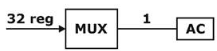# GATE Solved Paper 2020 - GATE 2020

• A

0.125• B

0.102• C

0.215• D

0.152• Option : A
• Explanation :
No. of relation on A = 29
No. of reflexive relation on A = 2(n2-n) = 2(32-3) = 26
∴ Probability (reflexive) = 26 / 29 = 1/8 = 0.125

• A

13• B

13.5• C

12• D

12.5• Option : B
• Explanation :
Cache Memory = 1 MB
Word size = 64 bit = 8 B
Block size = 256 B
Hit rate, x = 0.94 miss rate= 1-x = 0.06
Cache access time, Tc = 3ns
Number of words / Block = 256/8 = 32
Using Hierarchical Approach,
Tavg= ( x.Tc ) + (1-x)[ Tc + 1st word access time + remaining word access time]
Tavg = (0.94 × 3) + (1 – 0.94) [3 + 20 + (31 × 5)]
Tavg = 13.5 ns

• A

6• B

7• C

8• D

4• Option : B
• Explanation :
S → aSB
→ aaSBB [S → aSB]
→ aaaSBBB [S → aSB]
Total 7 steps require

• A

1024• B

10• C

1030• D

1034• Option : D
• Explanation :
⇒ 210 bytes
m = 10
n = 2m
m = 10, n = 1024
so, m+n= 1024+ 10 = 1034

• A

5• B

4• C

6• D

3• Option : A
• Explanation :MUX: 2n inputs, n selection lines, 1 o/p
2n = 32 ⇒ n = 5
Related Quiz.
GATE 2020